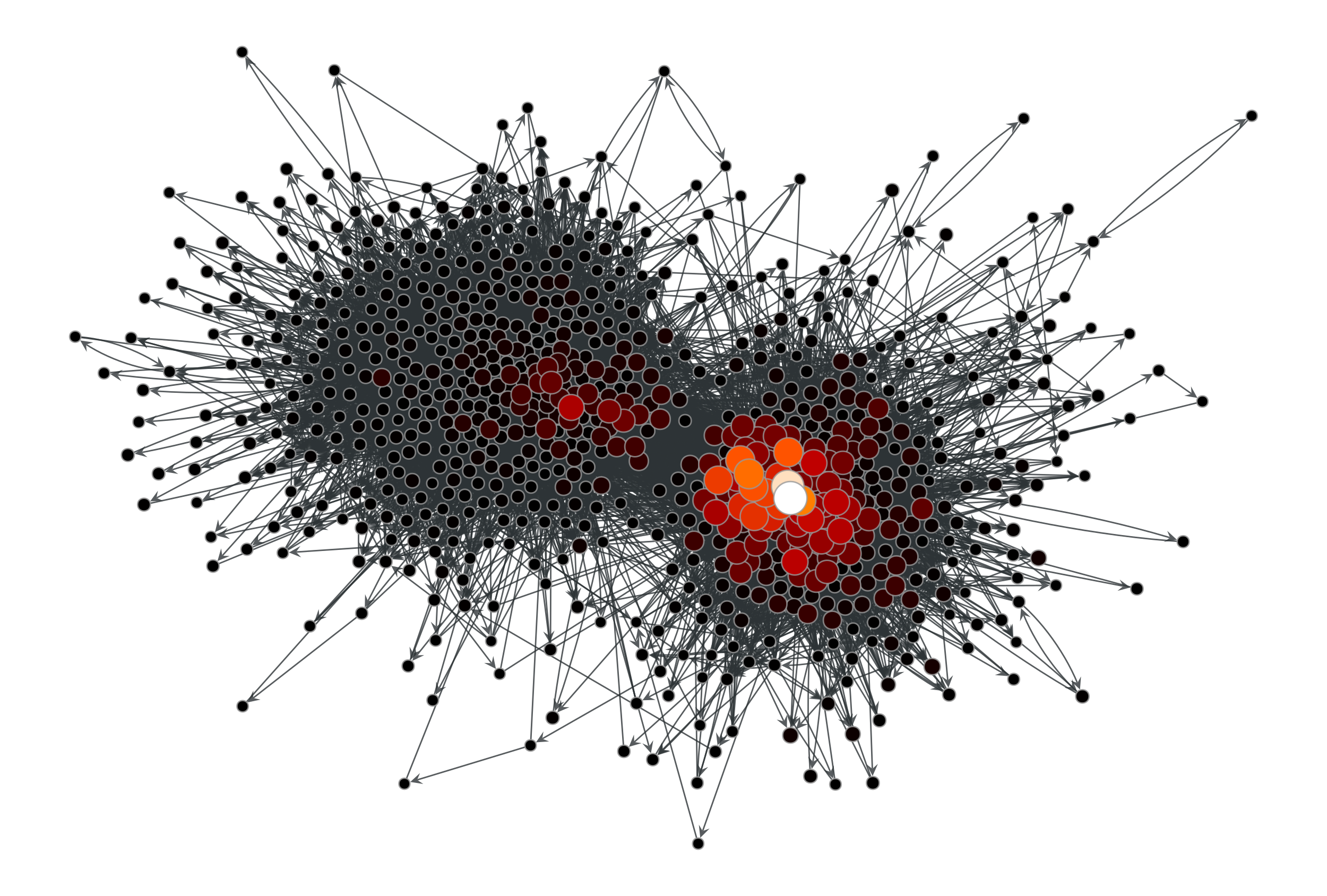# graph_tool.centrality.eigenvector#

graph_tool.centrality.eigenvector(g, weight=None, vprop=None, epsilon=1e-06, max_iter=None)[source]#

Calculate the eigenvector centrality of each vertex in the graph, as well as the largest eigenvalue.

Parameters:
gGraph

Graph to be used.

weightEdgePropertyMap (optional, default: None)

Edge property map with the edge weights.

vpropVertexPropertyMap, optional (default: None)

Vertex property map where the values of eigenvector must be stored. If provided, it will be used uninitialized.

epsilonfloat, optional (default: 1e-6)

Convergence condition. The iteration will stop if the total delta of all vertices are below this value.

max_iterint, optional (default: None)

If supplied, this will limit the total number of iterations.

Returns:
eigenvaluefloat

The largest eigenvalue of the (weighted) adjacency matrix.

eigenvectorVertexPropertyMap

A vertex property map containing the eigenvector values.

betweenness

betweenness centrality

pagerank

PageRank centrality

hits

authority and hub centralities

trust_transitivity

pervasive trust transitivity

Notes

The eigenvector centrality $$\mathbf{x}$$ is the eigenvector of the (weighted) adjacency matrix with the largest eigenvalue $$\lambda$$, i.e. it is the solution of

$\mathbf{A}\mathbf{x} = \lambda\mathbf{x},$

where $$\mathbf{A}$$ is the (weighted) adjacency matrix and $$\lambda$$ is the largest eigenvalue.

The algorithm uses the power method which has a topology-dependent complexity of $$O\left(N\times\frac{-\log\epsilon}{\log|\lambda_1/\lambda_2|}\right)$$, where $$N$$ is the number of vertices, $$\epsilon$$ is the epsilon parameter, and $$\lambda_1$$ and $$\lambda_2$$ are the largest and second largest eigenvalues of the (weighted) adjacency matrix, respectively.

If enabled during compilation, this algorithm runs in parallel.

References

[langville-survey-2005]

A. N. Langville, C. D. Meyer, “A Survey of Eigenvector Methods for Web Information Retrieval”, SIAM Review, vol. 47, no. 1, pp. 135-161, 2005, DOI: 10.1137/S0036144503424786 [sci-hub, @tor]

L. A. Adamic and N. Glance, “The political blogosphere and the 2004 US Election”, in Proceedings of the WWW-2005 Workshop on the Weblogging Ecosystem (2005). DOI: 10.1145/1134271.1134277 [sci-hub, @tor]

Examples

>>> g = gt.collection.data["polblogs"]
>>> g = gt.GraphView(g, vfilt=gt.label_largest_component(g))
>>> w = g.new_edge_property("double")
>>> w.a = np.random.random(len(w.a)) * 42
>>> ee, x = gt.eigenvector(g, w)
>>> gt.graph_draw(g, pos=g.vp["pos"], vertex_fill_color=x,
...               vertex_size=gt.prop_to_size(x, mi=5, ma=15),
...               vcmap=matplotlib.cm.gist_heat,
...               vorder=x, output="polblogs_eigenvector.pdf")
<...>Eigenvector values of the a political blogs network of [adamic-polblogs], with random weights attributed to the edges.#